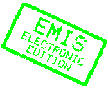MATHEMATICA BOHEMICA, Vol. 130, No. 2, pp. 203-220 (2005)

# Generalized $F$-semigroups

## E. Giraldes, P. Marques-Smith, H. Mitsch

Emilia Giraldes, UTAD, Dpto. de Matematica, Quinta de Prados, 5000 Vila Real, Portugal; Paula Marques-Smith, Universidade do Minho, Centro de Matematica, Campus de Gualtar, 4700 Braga, Portugal; Heinz Mitsch, Universit\"{a}t Wien, Institut f\"{u}r Mathematik, Nordbergstrasse 15, 1090 Wien, Austria, e-mail: heinz.mitsch@univie.ac.atAbstract: A semigroup $S$ is called a generalized $F$- semigroup if there exists a group congruence on $S$ such that the identity class contains a greatest element with respect to the natural partial order $\leq_{S}$ of $S$. Using the concept of an anticone, all partially ordered groups which are epimorphic images of a semigroup $(S,\cdot,\leq_{S})$ are determined. It is shown that a semigroup $S$ is a generalized $F$-semigroup if and only if $S$ contains an anticone, which is a principal order ideal of $(S,\leq_{S})$. Also a characterization by means of the structure of the set of idempotents or by the existence of a particular element in $S$ is given. The generalized $F$-semigroups in the following classes are described: monoids, semigroups with zero, trivially ordered semigroups, regular semigroups, bands, inverse semigroups, Clifford semigroups, inflations of semigroups, and strong semilattices of monoids.

Keywords: semigroup, natural partial order, group congruence, anticone, pivot

Classification (MSC2000): 20M10

Full text of the article:

[Previous Article] [Contents of this Number] [Journals Homepage]
© 2005–2010 FIZ Karlsruhe / Zentralblatt MATH for the EMIS Electronic Edition